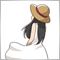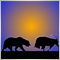Difference of two indicators30

Hi,

I want to get one indicator which is the difference of two other indicators in an indicator window. For instance MA10 and MA20.

Is this possible easy or must it be coded?

thanks

regards475

waterdevil:

Hi,

I want to get one indicator which is the difference of two other indicators in an indicator window. For instance MA10 and MA20.

Is this possible easy or must it be coded?

thanks

regards

MACD show us the difference of two MA.30

MACD show us the difference of two MA.

OK, yes I know, bad example. What if I want to add or subtract or average of ATR with CCI. Nevertheless if this has sense wit ATR,CCI. There are pairs with sense.475

It is possible to add values ​​such as moving average, RSI, CCI etc to one indicator.
Simply drop it to your indicator to which you want to add it and apply Prevous Indicator's Data or First Indicator's Data to the applied price.
However, there is no way to calculate the difference between the value of two indicators.293

It is possible to add values ​​such as moving average, RSI, CCI etc to one indicator.
Simply drop it to your indicator to which you want to add it and apply Prevous Indicator's Data or First Indicator's Data to the applied price.
However, there is no way to calculate the difference between the value of two indicators.
I thought, if I do this, then the dropped MA is MA from first MA and not added.1201

Hi, there's a way to do what you want: transform your indicators into percentage and then do whatever you want with these indicator (that now have values that are scaled by %).

Here is the formula to do that, for example with CCI (you have to calculate 3 values, MAX, MIN, AVERAGE of MAX and MIN):

(CCI value - AVERAGE of (MAX of 1000 past CCI data AND MIN of 1000 past CCI data ) / MAX of 1000 CCI data - AVERAGE)*100

Do the same with another indicator, for example RSI...now you can also display the two indicator on the same chart, cause now they have values scaled in the same way.

I've never tried this on Metatrader, but I'm sure it works cause I used it on many indicators that I made in Excel years ago.